# AP Calculus BC Practice Test 35

### Test Information8 questions24 minutes

Calculator Allowed

1. If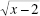is replaced by u, then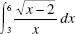is equivalent to

2. The set of all x for which the power series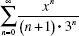converges is

3. A particle moves along a line with acceleration a = 6t. If, when t = 0, v = 1, then the total distance traveled between t = 0 and t = 3 equals

4. The definite integral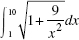represents the length of an arc. If one end of the arc is at the point (1,2), thenan equation describing the curve is

5. Suppose f (3) = 2, f (3) = 5, and f (3) = -2. Then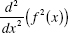at x = 3 is equal to

6. Which statement is true?

7.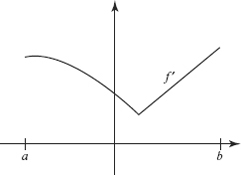The graph of f is shown above. Which statements about f must be true for a <x < b ?

I. f is increasing.

II. f is continuous.

III. f is differentiable.

8. After a bomb explodes, pieces can be found scattered around the center of the blast.The density of bomb fragments lying x meters from ground zero is given by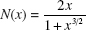fragments per square meter. How many fragments will be found within 20 meters ofthe point where the bomb exploded?